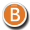• 3D Figure Assets

By:  Deva3D ()

7.95 USD
50% off• 3D Figure Assets

By:  Deva3D ()

15.50 USD• 3D Figure Assets

By:  Deva3D ()

7.75 USD
50% off• 3D Figure Assets

By:  Deva3D ()

15.90 USD• 3D Figure Assets

By:  Deva3D ()

15.90 USD• 3D Figure Assets

By:  Deva3D ()

8.45 USD
50% off• 3D Figure Assets

By:  Deva3D ()

15.50 USD• 3D Figure Assets

By:  Deva3D ()

16.90 USD• 3D Figure Assets

By:  Deva3D ()

7.95 USD
50% off• 3D Figure Assets

By:  Deva3D ()

7.95 USD
50% off• 3D Figure Assets

By:  Deva3D ()

15.90 USD• 3D Figure Assets

By:  Deva3D ()

15.50 USD• 3D Figure Assets

By:  Deva3D ()

15.50 USD• 3D Figure Assets

By:  Deva3D ()

7.75 USD
50% off• 3D Figure Assets

By:  Deva3D ()

15.90 USD• 3D Figure Assets

By:  Deva3D ()

6.36 USD
60% off• 3D Figure Assets

By:  Deva3D ()

15.90 USD• 3D Figure Assets

By:  Deva3D ()

16.90 USD• 3D Figure Assets

By:  Deva3D ()

17.50 USD• 3D Figure Assets

By:  Deva3D ()

15.90 USD• 3D Figure Assets

By:  Deva3D ()

15.90 USD• 3D Figure Assets

By:  Deva3D ()

15.90 USD• 3D Figure Assets

By:  Deva3D ()

15.90 USD• 3D Figure Assets

By:  Deva3D ()

15.90 USD• 3D Figure Assets

By:  Deva3D ()

15.90 USD• 3D Figure Assets

By:  Deva3D ()

15.90 USD• 3D Figure Assets

By:  Deva3D ()

15.90 USD• 3D Figure Assets

By:  Deva3D ()

15.50 USD• 3D Figure Assets

By:  Deva3D ()

16.49 USD• 3D Figure Assets

By:  Deva3D ()

14.90 USD• 3D Figure Assets

By:  Deva3D ()

16.49 USD• 3D Figure Assets

By:  Deva3D ()

16.49 USD• 3D Figure Assets

By:  Deva3D ()

16.49 USD• 3D Figure Assets

By:  Deva3D ()

16.49 USD• 3D Figure Assets

By:  Deva3D ()

14.50 USD• 3D Figure Assets

By:  Deva3D ()

14.50 USD• 3D Figure Assets

By:  Deva3D ()

15.50 USD• 3D Figure Assets

By:  Deva3D ()

15.90 USD• 3D Figure Assets

By:  Deva3D ()

15.90 USD• 3D Figure Assets

By:  Deva3D ()

14.50 USD• 3D Figure Assets

By:  Deva3D ()

14.50 USD• 3D Figure Assets

By:  Deva3D ()

14.50 USD• 3D Figure Assets

By:  Deva3D ()

14.50 USD• 3D Figure Assets

By:  Deva3D ()

16.49 USD• 3D Figure Assets

By:  Deva3D ()

15.90 USD• 3D Figure Assets

By:  Deva3D ()

14.50 USD• 3D Figure Assets

By:  Deva3D ()

14.50 USD• 3D Figure Assets

By:  Deva3D ()

14.50 USD• 3D Figure Assets

By:  Deva3D ()

15.90 USD• 3D Figure Assets

By:  Deva3D ()

15.90 USD• 3D Figure Assets

By:  Deva3D ()

14.50 USD• 3D Figure Assets

By:  Deva3D ()

14.50 USD• 3D Figure Assets

By:  Deva3D ()

14.50 USD• 3D Figure Assets

By:  Deva3D ()

14.50 USD